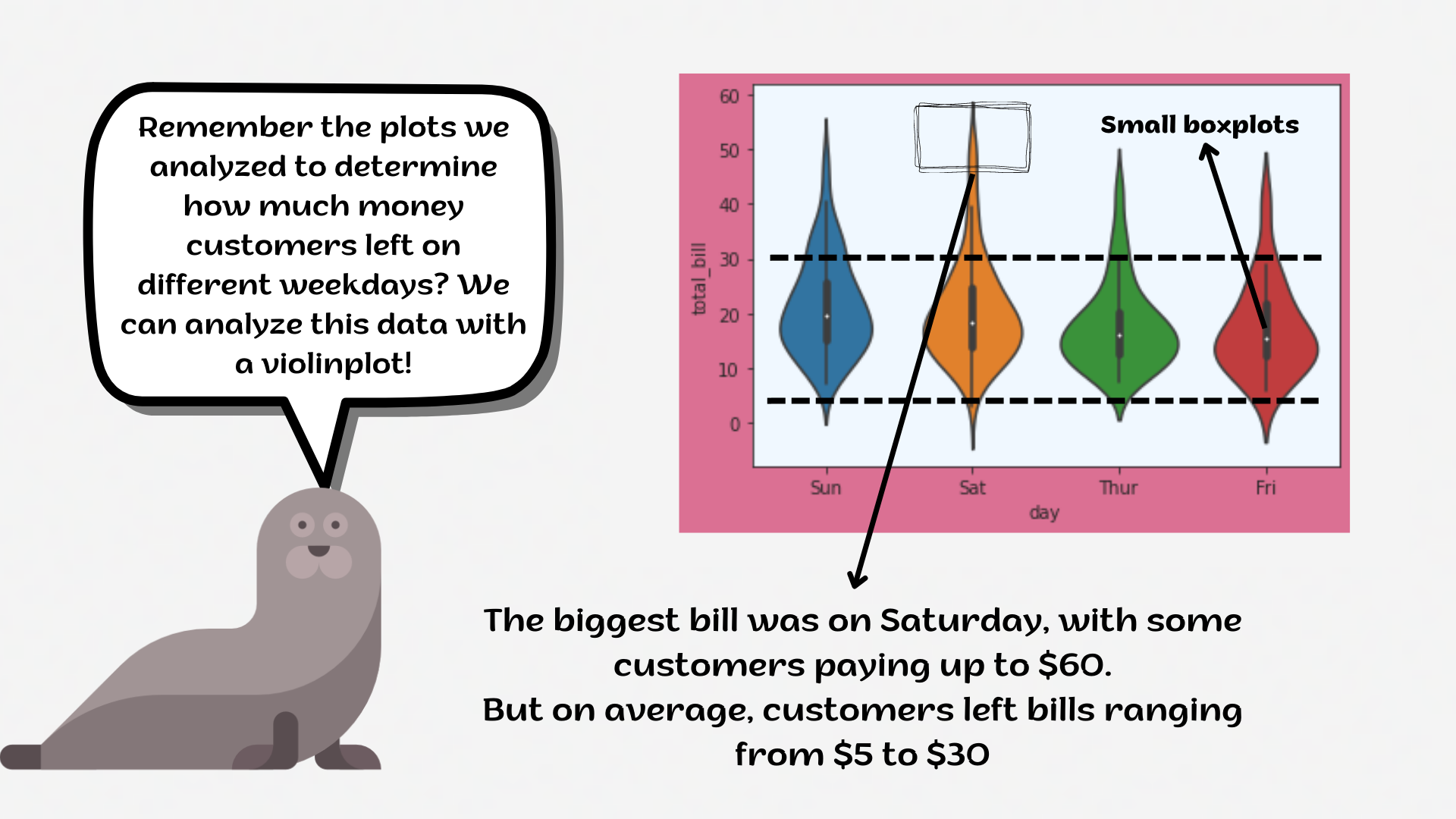Course Content

# Deep Dive into the seaborn Visualization

1. Light Start

2. Distributions of Data

3. Categorical Plot Types

4. Matrix Plots

5. Multi-Plot Grids

6. Regression Models

Deep Dive into the seaborn Visualization

##ViolinplotA `violinplot` is a hybrid of a box plot and a kernel density plot, which shows peaks in the data. It is used to visualize the distribution of numerical data. Unlike a box plot that can only show summary statistics, violin plots depict summary statistics and the density of each variable.1. Create a violinplot using the `g` variable:
• Set the `x` parameter equals the `'day'`;
• Set the `y` parameter equals the `'total_bill'`;
• Set the `hue` parameter equals `'sex'`;
• Set the `'rocket'` `palette`;
• Set the `split` parameter;
• Set the `inner` parameter equals the `'point'`;
• Set the `bw` parameter equals the `0.2`.
1. Set the title for the plot: `'Tips violinplot'`.

Everything was clear?

Section 3. Chapter 4